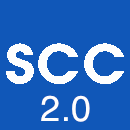# User's Guide for scc 2.0 (secondary cone cruiser)

scc is an implementation of Voronoi's reduction theory by Frank Vallentin and Achill Schürmann. It allows to cruise through the secondary cones of Delone triangulation (also known as L-type domains). See the paper "Computational approaches to lattice packing and covering problems" for a detailed description and applications to the construction of lattices with extremal properties. scc can in particular produce input files for coop and pacoop. Its new version, scc 2.0 by Alexey Garber, can also compute the list of all Delone decompositions, by cruising through all faces of full-dimensional secondary cones. The test whether or not two Delone decompositions are equivalent is now done by testing arithmetic equivalence of central reduced quadratic forms, using the program ISOM by Bernd Souvignier. As a consequence the program is much faster now and allows to obtain a complete list of 110244 inequivalent Delone decompositions in dimension 5. See the paper "The complete classification of five-dimensional Dirichlet-Voronoi polyhedra of translational lattices" for further information.

### USAGE:

```scc [options] < file
Options:
-h   show this help
-d D work in dimension D (required)
-a   find all Delone decompositions
-r N do a random walk of length N
-g   greedy approach
-w   write coop file for every secondary cone
-v   write central form information for every cone (only if -a option is chosen)
```

### EXAMPLES:

1. ```scc -d4

*** sc ***
***  c ***

Time: 19:37:47
Looking at #0
* Remaining: 0
* There are 10 neighbours.
* (0->1) (1=1) (2=1) (3=1) (4=1) (5=1) (6=1) (7=1) (8=1) (9=1)

Time: 19:37:47
Looking at #1
* Remaining: 0
* There are 10 neighbours.
* (0=1) (1=1) (2=1) (3=1) (4=1) (5=0) (6->2) (7=2) (8=2) (9=1)

Time: 19:37:47
Looking at #2
* Remaining: 0
* There are 10 neighbours.
* (0=1) (1=1) (2=1) (3=1) (4=1) (5=1) (6=1) (7=1) (8=2) (9=1)

--
```

2. ```scc -d4 -a

*** sc ***
***  c ***

Time: 19:28:39
Looking at #0
* Remaining: 0
* There are 10 neighbours.
* (0->1) (1=1) (2=1) (3=1) (4=1) (5=1) (6=1) (7=1) (8=1) (9=1)

Time: 19:28:39
Looking at #1
* Remaining: 0
* There are 10 neighbours.
* (0=1) (1=1) (2=1) (3=1) (4=1) (5=0) (6->2) (7=2) (8=2) (9=1)

Time: 19:28:39
Looking at #2
* Remaining: 0
* There are 10 neighbours.
* (0=1) (1=1) (2=1) (3=1) (4=1) (5=1) (6=1) (7=1) (8=2) (9=1)

--
Classification of Delone triangulations completed!
There are 3 non-equivalent Delone triangulations in dimension 4.

Starting classification of arbitrary Delone decompositions
There are 3 forms of codimension 0
Time: 19:28:39
There are 4 forms of codimension 1
Time: 19:28:39
There are 7 forms of codimension 2
Time: 19:28:39
There are 11 forms of codimension 3
Time: 19:28:39
There are 10 forms of codimension 4
Time: 19:28:39
There are 8 forms of codimension 5
Time: 19:28:39
There are 5 forms of codimension 6
Time: 19:28:39
There are 2 forms of codimension 7
Time: 19:28:39
There are 1 forms of codimension 8
Time: 19:28:39
There are 1 forms of codimension 9
Time: 19:28:39

Number of differrent decompositions: 52
*******
```

3. ```scc -d6 -r4 -w

*** sc ***
***  c ***

Time: 19:38:56
Looking at #0 (normalized covering density >= 0.493668)
* There are 21 neighbours.
* Write output for COOP
* Proceed with number 1.

Time: 19:38:56
Looking at #1 (normalized covering density >= 0.499392)
* There are 21 neighbours.
* Write output for COOP
* Proceed with number 4.

Time: 19:38:56
Looking at #2 (normalized covering density >= 0.502569)
* There are 21 neighbours.
* Write output for COOP
* Proceed with number 9.

Time: 19:38:57
Looking at #3 (normalized covering density >= 0.508167)
* There are 21 neighbours.
* Write output for COOP
* Proceed with number 19.
```

4. ```scc -d6 -g -i <sc6_3.coop

*** sc ***
***  c ***

Time: 19:51:13
Looking at #0 (normalized covering density >= 0.508167)
* There are 21 neighbours.

Time: 19:51:13
Looking at #1 (normalized covering density >= 0.502569)
* There are 21 neighbours.

Time: 19:51:14
Looking at #2 (normalized covering density >= 0.499392)
* There are 21 neighbours.

Time: 19:51:14
Looking at #3 (normalized covering density >= 0.493668)
* There are 21 neighbours.
```

### INTERPRETATION:

1. Classifies all secondary cones in dimension 4.
2. Classifies all secondary cones in dimension 4. After the classification of full dimensional secondary cones (Delone triangulations) their faces are classified as well (giving all Delone decompositions).
3. Takes a random walk of length 4 from Voronoi's first type and writes all found secondary cones into coop-format with file names sc6_0.coop ... sc6_3.coop
4. Reads sc6_3.coop and takes a greedy approach to find a local minimum of the lower bound.

### INSTALLATION:

To install scc you have to install GMP first. On a linux system this can usually be done by running apt-get install libgmp3-dev.

Then you have to install NTL (A Library for doing Number Theory) of Victor Shoup; you should allow GMP support in NTL, so you should configure it with NTL_GMP_LIP=on.

Then run make of scc. You may have to edit the variable CXXFlags in the Makefile so that it finds NTL.

Also you might need to remove the -m64 flag from the Makefile if you use non 64-bit architecture OS.